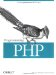14.13 Resources

 A resource is a generic data container that can hold any sort of data. An internal list mechanism keeps track of your resources, which are referenced through simple resource identifiers. Use resources in your extensions when the extension is providing an interface to something that needs cleanup. When the resource goes out of scope or your script ends, your destructor function for that resource is called, and you can free memory, close network connections, remove temporary files, etc. Here's a simple little example where we tie our resource to a trivial struct that contains only a string and an integer (name and age, in this case): static int le_test; typedef struct _test_le_struct { char *name; long age; } test_le_struct; The struct can contain anything: a file pointer, a database connection handle, etc. The destructor function for our resource looks like this: static void _php_free_test(zend_rsrc_list_entry *rsrc TSRMLS_DC) { test_le_struct *test_struct = (test_le_struct *)rsrc->ptr; efree(test_struct->name); efree(test_struct); } In your MINIT( ) function, add this line to register your destructor for the le_test resource: le_test = zend_register_list_destructors_ex(_php_free_test, NULL, "test", module_number); Now, here's a fictitious my_init( ) function that initializes the data associated with the resource. It takes a string and an integer (name and age): PHP_FUNCTION(my_init) { char *name = NULL; int name_len, age; test_le_struct *test_struct; if (zend_parse_parameters(ZEND_NUM_ARGS( ) TSRMLS_CC, "sl", &name, &name_len, &age) == FAILURE) { return; } test_struct = emalloc(sizeof(test_le_struct)); test_struct->name = estrndup(name, name_len); test_struct->age = age; ZEND_REGISTER_RESOURCE(return_value, test_struct, le_test); } And here's a my_get( ) function that takes a resource parameter returned from my_init( ) and uses that to look up the data associated with the resource: PHP_FUNCTION(my_get) { test_le_struct *test_struct; zval *res; if (zend_parse_parameters(ZEND_NUM_ARGS( ) TSRMLS_CC, "r", &res) == FAILURE) { return; } ZEND_FETCH_RESOURCE(test_struct, test_le_struct *, &res, -1, "test", le_test); if(!test_struct) RETURN_FALSE; array_init(return_value); add_assoc_string(return_value, "name", test_struct->name, 1); add_assoc_long(return_value, "age", test_struct->age); }Programming PHP
ISBN: 1565926102
EAN: 2147483647
Year: 2007
Pages: 168

Similar book on Amazon